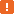# aqConvert.StrToFloat Method### Description

The `StrToFloat` method converts the string specified by the `S` parameter to a floating-point value.

### Declaration

aqConvert.StrToFloat(S)

 S [in] Required String Result Double

### Applies To

The method is applied to the following object:

### Parameters

The method has the following parameter:

S

The string to be converted to a number.

The string must be in the following format (square brackets [ ] denote optional elements; braces { } denote a required element; a vertical bar | separates alternatives):

[whitespace] [+|-] {digits | [digits].digits} [{E|e} [+|-] digits] [whitespace]

A valid string includes an optional sign (+ or -) followed by a series of digits with an optional decimal point.The decimal separator depends on the computer’s regional settings. For instance, the US English locale uses a period (.), while the Iceland locale uses a comma (,).

The number may be followed by an exponent consisting of the letter E or e and an optionally signed whole number. Leading and trailing whitespace characters are ignored. Thousand separators and currency symbols are not allowed in the string.

### Result Value

A floating-point number that is specified in the input string.

### Remarks

If the specified string cannot be converted to a number (for example, it has a wrong format), `StrToFloat` raises an exception with the following text: "The argument is not a number."

### Example

The code below demonstrates how you can convert strings to floating-point values.

 Note: This example assumes the US English locale or any other locale that uses a period (.) as the decimal separator.

JavaScript, JScript

function StringToFloat()
{
Log.Message(aqConvert.StrToFloat("14535.47"));
}

/*
The routine produces the following output:
14535.47
*/

Python

``````def StringToFloat():
Log.Message(aqConvert.StrToFloat("14535.47"))

# The routine produces the following output:
# 14535.47``````

VBScript

Sub StringToFloat
Log.Message(aqConvert.StrToFloat("14535.47"))
End Sub

' The routine produces the following output:
' 14535.47

DelphiScript

procedure StringToFloat;
begin
Log.Message(aqConvert.StrToFloat('14535.47'));
end;

{
The routine produces the following output:
14535.47
}

C++Script, C#Script

function StringToFloat()
{
Log["Message"](aqConvert["StrToFloat"]("14535.47"));
}

/*
The routine produces the following output:
14535.47
*/